Chapter 11: Functions

# 11.9 Your Next Year’s Valentine?

Fill in the data tables and draw the graphs for each. Use the suggested scaling for each axis.

1.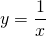Scaling: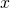-axis: 1 square = 1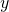-axis: 1 square = 110
8
5
4
2
1
0.5
0.25
0.2
0.125
0.1
2.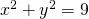Scaling:-axis: 2 squares = 1-axis: 2 squares = 13
2.5
2
1.5
1
0.5
0
−0.5
−1
−1.5
−2
−2.5
−3
3.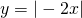Scaling:-axis: 1 square = 1-axis: 1 square = 15
4
3
2
1
0
−1
−2
−3
−4
−5
4.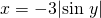Scaling:-axis: 2 squares = 1-axis: 1 square = 30°180°
150°
120°
90°
60°
30°
−30°
−60°
−90°
−120°
−150°
−180°